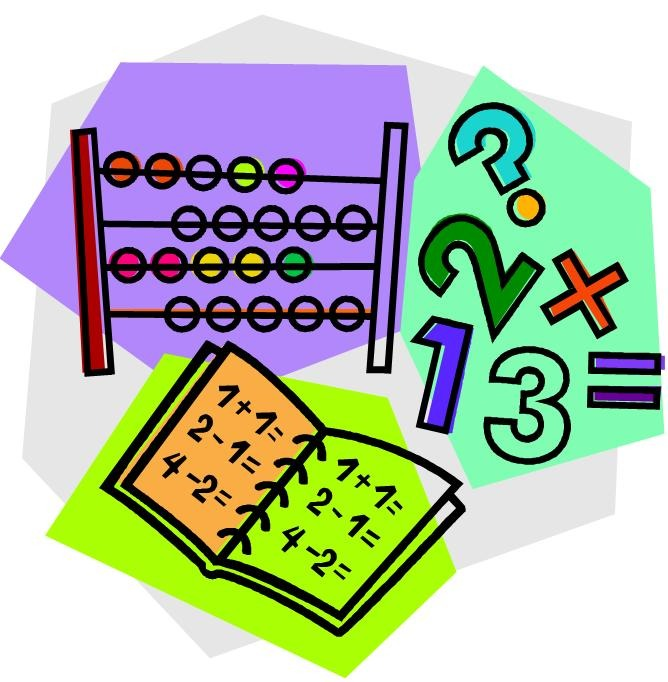Author:
Tina
Subject:
Secondary Mathematics
Material Type:
Lesson
Level:
Middle School, High School
Tags:
• Lesson Plan
Language:
English
Media Formats:
Text/HTML

# Solving Equations With Addition and Subtraction## Overview

This lesson introduces students to the basics of solving simple algebraic equations using addition and subtraction.

# Summary

This lesson introduces students to the basics of solving simple algebraic equations using addition and subtraction.

Thumbnail image courtesy of clipartpanda.com

# Background for Teachers

To teach this lesson, you will need an understanding of solving simple algebra equations using addition and subtraction.  See the video below if you need a review:

# Step 1 - Goals and Outcomes

Students will understand how to solve a simple algebraic equation with one variable using addition and subtract.  Students demonstrate their knowledge via assessment described below.

# Step 2 - Planning Instruction

When planning instruction consider the background knowledge of the students.  If numerical fluency will be a stumbling block, have the students use calculators.  Be sure to give all students a calculator so no one is singled out.  Students who struggle with handwriting may wish to use an electronic device for the assignment.

Virtual manipulatives may also be used to illustrate the concept.  If your computers will run Java the Algebra Balance Scale from nlvm.usu.edu correlates with this lesson.

# Step 3 - Instruction

1.  Have the students do the warmup as a bell-ringer activity.

2.  Have students take out their note-taking journals and a calculator (calculator for scaffolding).

3.  Go through the powerpoint.

4.  Have the students do the classwork activity individually as a formative assessment.  Observe the students and give individual help as needed.

5.  Go over the classwork activity with the students.

6.  Assign the homework as a summative assessent.

# Step 4 - Assessments

This lesson contains a pre-assessement (warmup activity), formative assessments during the powerpoint lesson and while observing the classwork activity, and a summative assessment (homework activity).  Use the attached rubric to grade the homework.